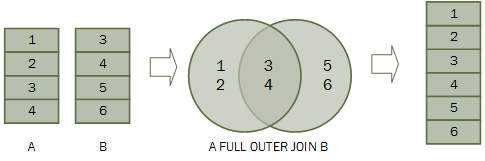# Explain the binary operations in relational algebra (DBMS)?

Query is a question or requesting information. Query language is a language which is used to retrieve information from a database.

Query language is divided into two types −

• Procedural language

• Non-procedural language

## Procedural language

Information is retrieved from the database by specifying the sequence of operations to be performed.

For Example − Relational algebra.

Structure Query language (SQL) is based on relational algebra.

Relational algebra consists of a set of operations that take one or two relations as an input and produces a new relation as output.

## Types of Relational Algebra operations

The different types of relational algebra operations are as follows −

• Select operation

• Project operation

• Rename operation

• Union operation

• Intersection operation

• Difference operation

• Cartesian product operation

• Join operation

• Division operation

Union, intersection, difference, cartesian, join, division comes under binary operation (operate on two tables).

## Union operation

Union operation combines the values in R1, R2 removing duplicate ones.

### Syntax

The syntax for the union operation is as follows −

∏ regno(R1) ∪ ∏ regno(R2)

It displays all the regno of R1 and R2.

### Example

Consider two tables R1 and R2 as shown below −

### Table R1

RegnoBranchSection
1CSEA
2ECEB
3MECHB
4CIVILA
5CSEB

### Table R2

RegnoBranchSection
1CIVILA
2CSEA
3ECEB

To display all the regno of R1 and R2, use the following command −

∏ regno(R1) ∪ ∏ regno(R2)

### Output

Regno
1
2
3
4
5

No replacement is allowed for the entry.

Union All is used for the duplicate entries.

Common entries will be shown if a common intersection is used.

## Intersection operation

It displays the common values in R1 & R2. It is denoted by ∩.

The syntax for the intersection operation is as follows −

∏ regno(R1) ∩ ∏ regno(R2)

### Example 1

Consider two sets,

A={1,2,4,6} and B={1,2,7}

Intersection of A and B

A ∩ B ={1,2}

Elements that are present in both sets A and B are present in the set obtained by intersection of A and B.

In relational algebra if R1 and R2 are two instances of relation then,

R1 ∩ R2 ={ x | x€ R1 and x € R2}

That is, at the intersection of R1 and R2 only those tuples will be present that are in both R1 and R2.

### Example 2

Find all the customers whose account is in the bank and they have taken out a loan.

The expression will be as follows −

∏Name(Depositor) ∩ ∏Name(Borrower)

IDName
1A
2B
3C

### Borrower

IDName
2B
3A
5D

So intersection of Depositor and borrower is as follows −

 A B

## Cartesian product operation

It combines R1 and R2 without any condition.

It is denoted by X.

Degree of R1 XR2 = degree of R1 + degree of R2

{degree = total no of columns}

### Example

Consider R1 table as given below −

RegNoBranchSection
1CSEA
2ECEB
3CIVILA
4ITB

Table R2 is as follows −

NameRegNo
BhanuPriya
24

Therefore, the result of R1 X R2 is as follows −

RegNoBranchSectionNameRegNo
1CSEABhanu2
1CSEAPriya4
2ECEBBhanu2
2ECEBPriya4
3CIVILABhanu2
3CIVILAPriya4
4ITBBhanu2
4ITBPriya4

## Join operation

It combines the relation R1 and R2 with respect to a condition. It is denoted by ⋈.

The different types of join operation are as follows −

• Theta join

• Natural join

• Outer join

• Outer join

• Left outer join.

• Right outer join.

• Full outer join.

## Theta join

If we join R1 and R2 other than the equal condition, then it is called theta join/ non-equi join.

### Example

Consider R1 table

RegNoBranchSection
1CSEA
2ECEB
3CIVILA
4ITB
5ITA

### Table R2

NameRegNo
Bhanu2
Priya4

R1 ⋈ R2 with condition R1.regno > R2.regno

RegNoBranchSectionNameRegno
3CIVILABhanu2
4ITBBhanu2
5ITABhanu2
5ITBPriya4

In the join operation, we select those rows from the cartesian product where R1.regno>R2.regno.

Join operation = select operation + cartesian product operation

### Natural join

If we join R1 and R2 on equal condition then it is called natural join or equi join. Generally, join is referred to as natural join.

Natural join of R1 and R2 is as follows −

{we select those tuples from cartesian product where R1.regno=R2.regno}

R1 ⋈ R2

RegnoBranchSectionName
2--Bhanu
4--Priya

## Outer join

It is an extension of natural join to deal with missing values of relation.

Consider R1 and R2 shown below −

### Table R1

RegnoBranchSection
1CSEA
2ECEB
3CIVILA
4ITB
5ITA

### Table R2

NameRegno
Bhanu2
Priya4
Hari7

Outer join is of three types. These are explained below with examples.

### Left outer join

It is denoted by R1 ⋈ R2

RegnoBranchSectionNameRegno
2--Bhanu2
4--Priya4
1--NULLNULL
3--NULLNULL
5--NULLNULL

Here all the tuples of R1(left table) appear in output.

The mismatching values of R2 are filled with NULL.

Left outer join = natural join + mismatch / extra tuple of R1.

### Right outer join

It is denoted by R1 ⋈ R2

Here all the tuples of R2(right table) appear in output. The mismatching values of R1 are filled with NULL.

RegnoBranchSectionNameRegno
2--Bhanu2
4--Priya4
NULLNULLNULLHari7

Right outer join = natural join+ mismatch/extra tuple of R2.

### Full outer join

It is denoted by R1 ⋈ R2.

Full outer join=left outer join U right outer join.

RegnoBranchSectionNameRegno
2--Bhanu2
4--Priya4
1--NULLNULL
3--NULLNULL
5--NULLNULL
NULLNULLNULLHari7

### Example

The diagram given below depicts the full outer join −## Division operation

The division operator is used for queries which involve the ‘all’.

R1 ÷ R2 = tuples of R1 associated with all tuples of R2.

### Example

Retrieve the name of subject that is taught in all courses

NameCourse
SystemBtech
Database
DatabaseBtech
AlgebraBtech
Course
Btech
Btech

÷

Name
database

=

The resulting operation must have all combinations of tuples of relation S that are present in the first relation or R.